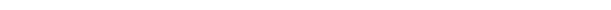Home DATA STRUCTURE Data Structure: What is Array?

# Data Structure: What is Array?

495
0#### Data Structure: What is Array?

An array is a Data Structure comprised of a group of elements (values or variables), each recognized by at least one array index or key. It is a collection of homogeneous items, i.e. it stores data elements having sane data type. It stores elements in contiguous memory locations.

• A particular element can be accessed by an index.
• The area of each element can be computed from its index tuple by a mathematical formula.
• The idea is to store multiple items of the same type together.
• Easier way to calculate the position of each element by simply adding an offset to a base value.
 element element element element element

Here element is the first element and element is the last element.

Declaring Array
In C programming we can declare a single dimensional array as follows:

 type array_name [ array_size ];

Note-
array_size must be a positive integer greater than zero.

Example-

 Int rollno;

Here rollno is a variable array that can hold 20 integer numbers.

Initializing Array

 Int rollno = {1, 2, 3, 4, 5 };

As we have specified the array limit as 5 enclosed in [ ], we can only have 5 elements in the array i.e. in { }.

If we keep the [ ] empty, it can hold a large number of elements.

 Int rollno[ ] = {1, 2, 3};

We can also initialize a single element at a time.

 Int rollno = 4;